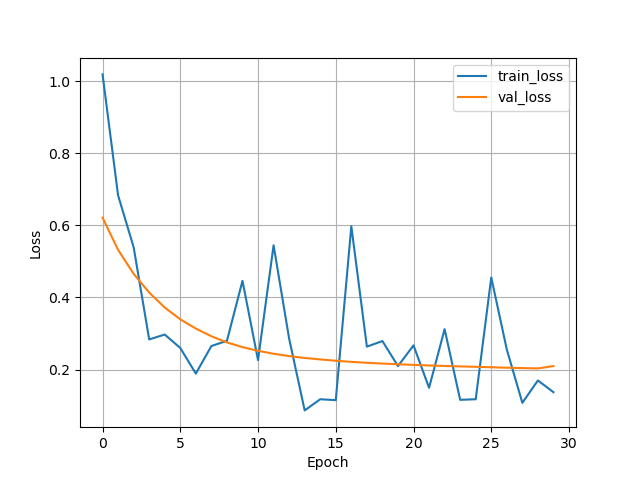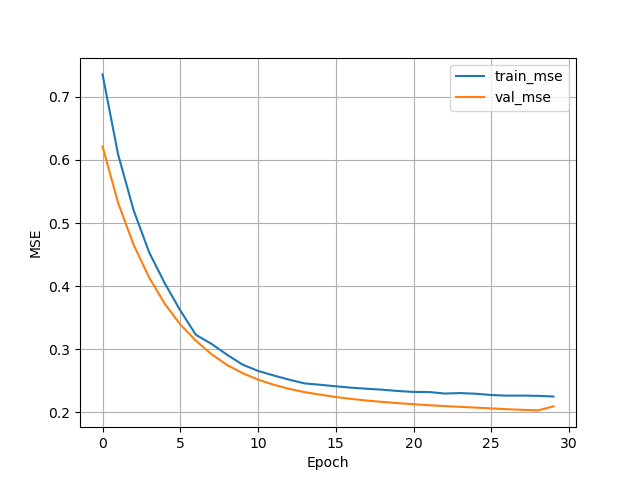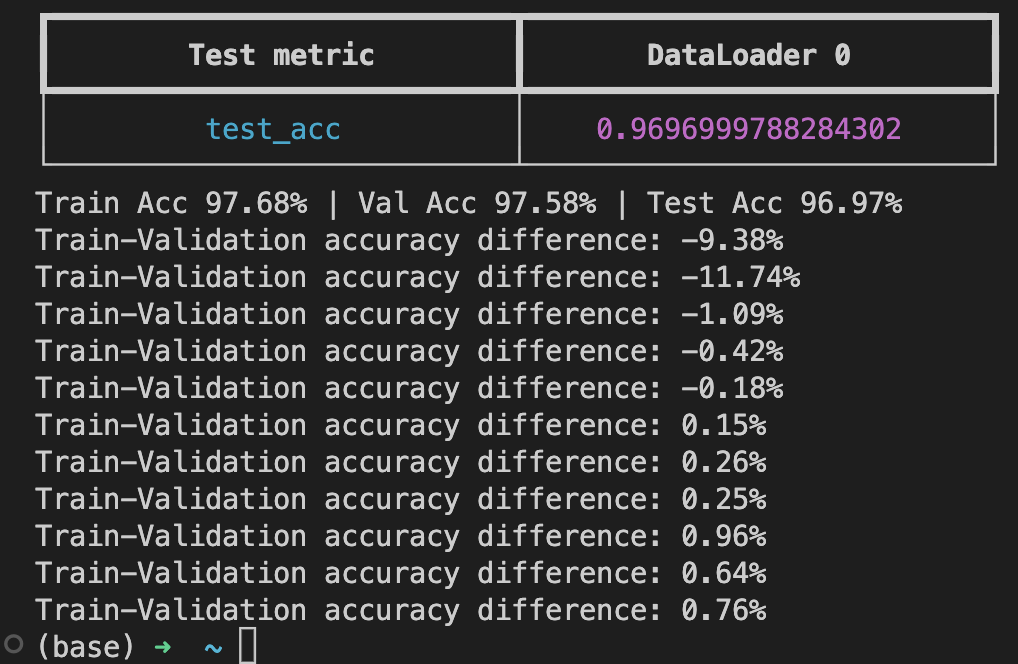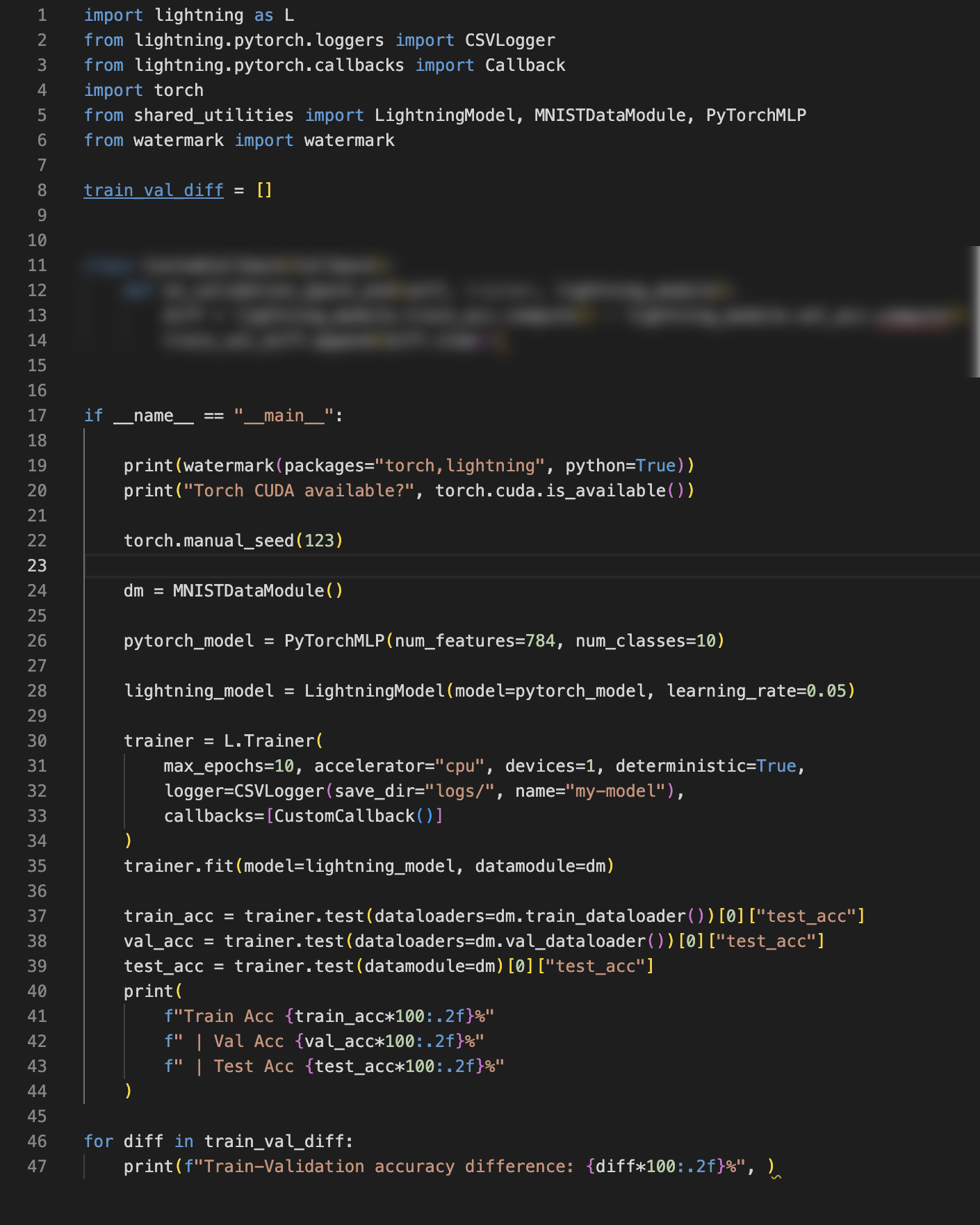Introducing Lit-LLaMA: a minimal, optimized rewrite of LLaMA licensed under Apache 2.0 →

# Exercise 1 – Changing the Classifier to a Regression Model

Remember the regression model we trained in Unit 4.5? To get some hands-on practice with PyTorch and the LightningModule class, we are going to convert the MNIST classifier we used in this unit (Unit 5) and convert it to a regression model.

For this, we are going to use the Ames Housing Dataset from OpenML. But no worries, you don’t have to code the Dataset and DataModule classes yourself! I already took care of that and provide you with a working AmesHousingDataModule. However your task is to change the PyTorchMLP into a regression model, and you have to make adjustments to the LightningModel to track the mean squared error instead of the classification accuracy.

If you are successful, the mean squared errors for the training, validation, and test set should be around 0.2 to 0.3.Here is a link to the start code: https://github.com/Lightning-AI/dl-fundamentals/tree/main/unit05-lightning/exercises/1_lightning-regression

And below are some tips and comments about changes you need to make.

As always, please don’t hesitate to reach out for help via the forums if you get stuck!

## In training.py

• UseTensorBoard or import the CSVLogger and use it in the Trainer (see Unit 6)
• Replace the MNISTDataModule() with the AmesHousingDataModule()(already provided in the shared_utilities.py file)
• Change the number of features in the PyTorchMLP(...) class to 3, remove the classes
• Change “acc” to “mse”
• Add the logging code at the bottom if you use the CSV logger. In that case, it would be:
metrics = pd.read_csv(f"{trainer.logger.log_dir}/metrics.csv")

aggreg_metrics = []
agg_col = "epoch"
for i, dfg in metrics.groupby(agg_col):
agg = dict(dfg.mean())
agg[agg_col] = i
aggreg_metrics.append(agg)

df_metrics = pd.DataFrame(aggreg_metrics)
df_metrics[["train_loss", "val_loss"]].plot(
grid=True, legend=True, xlabel="Epoch", ylabel="Loss"
)
df_metrics[["train_mse", "val_mse"]].plot(
grid=True, legend=True, xlabel="Epoch", ylabel="MSE"
)

plt.show()

## In shared_utilities.py

Modify the PyTorchMLP class as follows:

• Remove the num_classes argument from the PyTorchMLP
• Change the network to 1 output node & flatten the logits, (e.g,. using the torch.flatten function)
• Optionally only use 1 instead of 2 hidden layers to reduce overfitting

Modify the LightningModel class as follows:

• Use MeanSquaredError instead of Accuracy via torchmetrics
• Change the loss function accordingly
• Make sure you are returning the predictions correctly in _shared_step, they are not class labels anymore
• Change acc to mse` everywhere

# Exercise 2 – A Custom Plugin for Tracking Training and Validation Accuracy Difference

In this exercise, modify the existing MNIST classifier such that it tracks the difference between the training set and validation set accuracy after each epoch:Here, it’s easiest to implement the plug in such that it computers the training set accuracy minus the validation accuracy after each epoch ends. This difference is then added to a list that you can print at the end of your script. The solution for this exercise involves only a few lines of code as hinted add in the screenshot of the solution below:(Note that you could also compute these differences using the logfiles. However, this exercises aims to be an exercise practicing developing custom callbacks.)

Here is a link to the start code: https://github.com/Lightning-AI/dl-fundamentals/tree/main/unit05-lightning/exercises/2_custom-callback

As always, please don’t hesitate to reach out for help via the forums if you get stuck!